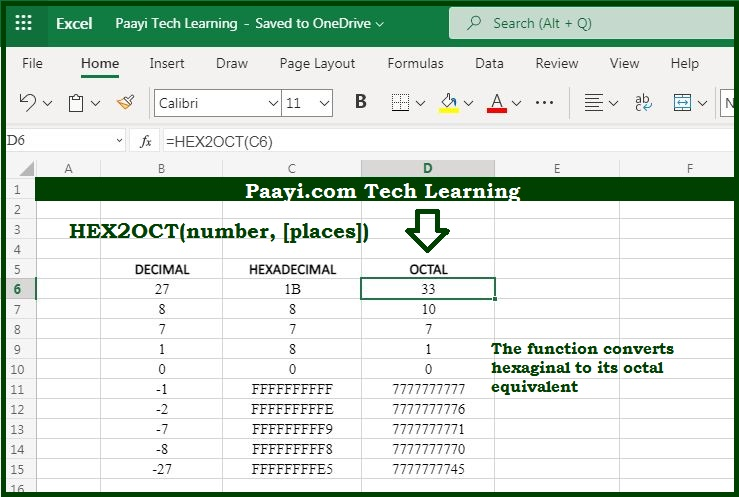# Learn How to Use Microsoft Excel HEX2OCT Function

Written by | 0 Comments | 673 Views

In this article, you will learn how to use the Microsoft Excel HEX2OCT function and its prime function in Microsoft Excel. You will also get to know the Microsoft Excel HEX2OCT function return value and syntax with the help of some examples.

Microsoft Excel HEX2OCT Function

The prime function of the Microsoft Excel HEX2OCT function is to convert hexadecimal numbers to octal numbers. That implies with the help of the HEX2OCT function you can able to convert the hexadecimal number to its octal equivalent.

Return Value of HEX2OCT Function

The return value will be the octal number.

Syntax of HEX2OCT Function

=HEX2OCT(number, [places])

Where the arguments:

number: It is the hexadecimal number that you want to convert into octal.

places: Joins the resulting octal number with zeros up to the specified number of digits. In case omitted returns the least number of characters required to represent the number (optional).

## How to Use Microsoft Excel HEX2OCT Function?As we know that HEX2OCT function used to convert hexadecimal numbers to their octal equivalent. Always keep in mind that:

The Microsoft Excel only converts to octal numbers of 10 digits or less, so the input range is restricted to 0 to 7777777777 octal numbers.

The input number should be less than or equal to the ten alphanumeric characters, or the function will return the error as #NUM!.

The internal (octal) representation of the hexadecimal number uses two's complement notation. The first bit shows whether the number is positive or negative, and the rest 39 bits denote the magnitude of the number.